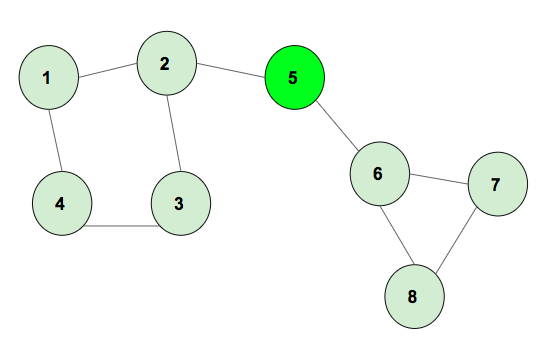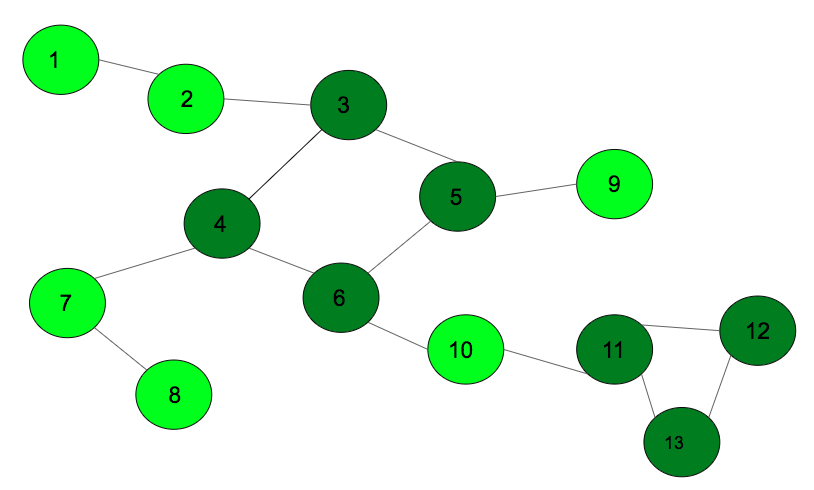Product of lengths of all cycles in an undirected graph

• Difficulty Level : Hard
• Last Updated : 07 Dec, 2021

Given an undirected and unweighted graph. The task is to find the product of the lengths of all cycles formed in it.
Example 1:The above graph has two cycles of length 4 and 3, the product of cycle lengths is 12.

Example 2:The above graph has two cycles of length 4 and 3, the product of cycle lengths is 12.

Approach: Using the graph coloring method, mark all the vertex of the different cycles with unique numbers. Once the graph traversal is completed, push all the similar marked numbers to an adjacency list and print the adjacency list accordingly. Given below is the algorithm:

• Insert the edges into an adjacency list.
• Call the DFS function which uses the coloring method to mark the vertex.
• Whenever there is a partially visited vertex, backtrack till the current vertex is reached and mark all of them with cycle numbers. Once all the vertexes are marked, increase the cycle number.
• Once Dfs is completed, iterate for the edges and push the same marked number edges to another adjacency list.
• Iterate in the another adjacency list, and keep the count of number of vertex in a cycle using map and cycle numbers
• Iterate for cycle numbers, and multiply the lengths to get the final product which will be the answer.

Below is the implementation of the above approach:

Javascript



Output:

12

Time Complexity: O(N), where N is the number of nodes in the graph.

My Personal Notes arrow_drop_up
Recommended Articles
Page :